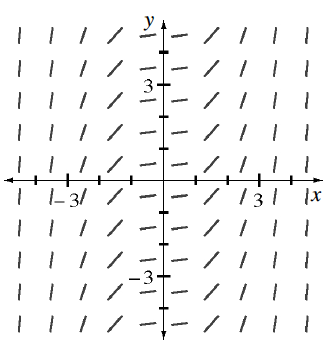### Home > APCALC > Chapter 7 > Lesson 7.3.5 > Problem7-157

7-157.

Solve for $y$ if $\frac { d y } { d x }= 0.5x^2$.

Then use the slope field for $\frac { d y } { d x }$ at right to help graph a family of solution functions (place your paper over the slope field and use the tangents as guides).

By tracing a series of slopes, you should predict that the original function is cubic.

Separate the variables so that $x$-values are on one side of the equal sign and $y$-values are on the other side.

$2dy = x^2dx$

Integrate both sides of the equation.

$\int 2dy=\int x^{2}dx$

$2y+C_{1}=\frac{x^{3}}{3}+C_{2}$

Solve for $y$. Did you get a cubic function, as predicted?
Note: $C_1 + C_2 = C_3$, or just $C$.